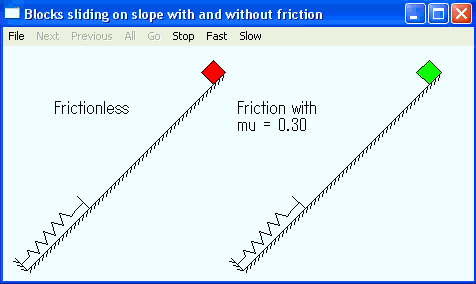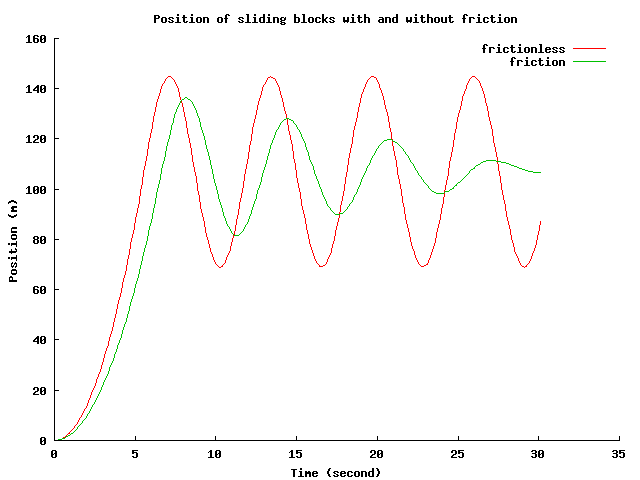Ch QuickAnimation Demos Sliding of Two Blocks With and Without Friction and Hitting a Spring. Problem Statement: Two blocks slide on slopes with the length of 100 meters and incline of 45 degrees. They will kit a spring with the coefficient of 1. One slope has no friction and the other has the friction with the friction coefficient mu of 0.5. Plot the traveling distance of these two blocks versus time and animate their motions. Animation This program blocks_spring.ch uses Ch QuickAnimation to animate the motion of sliding blocks shown below.Plotting The above same program blocks_spring.ch uses Ch plotting capabilities to plot the traejctories of sliding blocks shown below